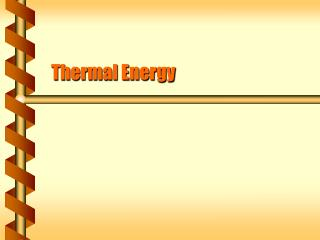DownloadDownload PresentationThermal Energy

# Thermal Energy

Télécharger la présentation## Thermal Energy

- - - - - - - - - - - - - - - - - - - - - - - - - - - E N D - - - - - - - - - - - - - - - - - - - - - - - - - - -
##### Presentation Transcript

1. Thermal Energy

2. Atomic Motion • The macroscopic properties of a system are based on the microscopic behavior of atoms. Macroscopic equation of state: pressure, volume, temperature Microscopic laws of motion: position, velocity, energy

3. Ideal Gas Energy • Kinetic theory supplies the energy for an ideal gas. • Kav = (3/2)kT • Convert this to the total system energy. • U = (3/2)NkT • U = (3/2)nRT • This energy is internal to the gas. Energy based on temperature and number of molecules

4. Internal Energy • The kinetic energy of an ideal gas is its internal energy. • Also called thermal energy • Internal energy does not include energy from external measures. • Center of mass translation • Rigid body rotation • Potential from external force vCM Fext

5. Vibrations • Mean particle speed is not the only source of internal energy. • Real molecules can have internal motion. • Rotation • Vibration • These effects add to internal energy. Rotation around center of mass Vibration at center of mass

6. Energy Transfer • Thermal energy changes in a system. • It flows in and out • Due to changes in macroscopic properties • When it is due to temperature changes it is called heat. Heat flow

7. Work is a process that represents the change in the mechanical energy. Force acting through a distance Measured in joules like energy Heat is a process that represents the change in the thermal energy. Due to a difference in temperature Measured in joules like energy Heat and Work

8. Mechanical Equivalent • In the 1800’s James Joule demonstrated that mechanical work could cause a change in temperature. • Heat, like work, represented a change in energy.

9. Heat was measured by a change in temperature. 1 calorie (cal) is the amount of heat needed to raise 1 g of water by 1 C. 1 kcal = 1000 cal, and is what we call a “Calorie” for food. Calories (or kcal) are often used to measure heat or internal energy. Since SI has a unit of energy (J) it remains the unit for all forms of energy. Work Mechanical energy Potential energy Dissipative energy Heat Thermal energy Internal energy 1 cal = 4.186 J Calorie

10. You gobble too much ice cream and decide to walk up stairs to burn the additional 500 Calories. If your mass is 60 kg, what vertical height must you climb? Convert to joules from kcal. W = (500 kcal)(1000 cal/kcal)(4.186 J/cal) W = 2.1 x 106 J. Work climbing is W = mgh. h = W / mg = (2.1 x 106 J) / (60 kg)(9.8 m/s2) h = 3600 m. Real humans are inefficient and h is closer to 700 m. Burn It next